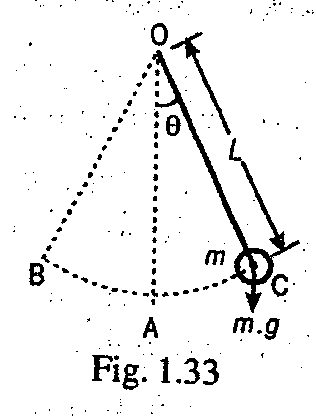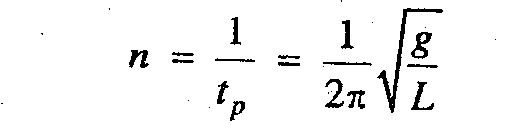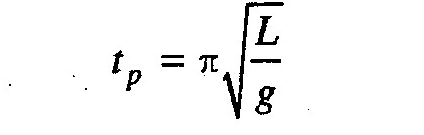# Simple PendulumA simple pendulum, in its simplest form, consists of a heavy bob suspended at the end of a light inextensible and flexible string as shown in Fig. 1.33. The other end of the string is fixed at O. When the bob is at A, it is in equilibrium or mean position. It may be noted that when the angle θ (through which the string is displaced) is less  than 4°, then the bob will oscillate between B and C with simple harmonic motion.
For a simple pendulum, periodic time,and frequency of oscillation,Notes:

1. The motion of the bob from one extremity to the other (i.e. from B to C or C to B) is known as beat or swing. Thus one beat = 1/2 oscillation, and periodic time for one beat,2. A pendulum, which executes one beat per second ( i.e. one complete oscillation in two seconds) is known as second's pendulum.